# Table factoral

Factorialan arbitrary integer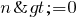is determined by the formula: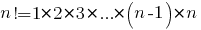By definition, the factorial of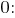Factorial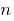equal to the number of permutations ofelements.

In combinatorics , the factorial of a natural numberis interpreted as the number of permutations (ordering) of the set withelements. For example, the set {A, B, C, D} of 4 elements there are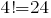changes:

## Table factoral numbers from 1 to 25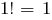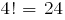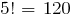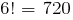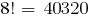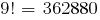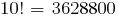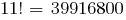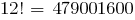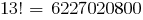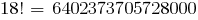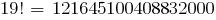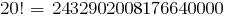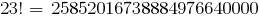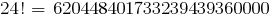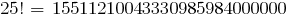Versions in other languages:
Share with friends: